## 2. 5. 1 Basis Operations

In the following, a basic calculus of operations is defined which is required to formulate a discrete problem in a functional manner: The calculation is defined exactly and can be carried out by a computer. At a first glance, such a formalism seems to be trivial due to the fact that each programming language defines basic arithmetic features. However, the handling of the underlying topological structures is not supported at all so that additional features have to be provided.

The most essential element of a description formalism is the access to a function which is defined on the cell complex. Due to data structural considerations, the domain of this function can be restricted to a certain skeleton of the underlying complex. However, this does not affect the concept of the function. In this context the definition of a quantity is employed in order to obtain the respective value for a given topological element. In the formalism such a function can be formulated as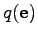, where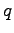and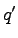denote the quantities and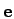denotes the element on which the quantities are evaluated.

In the next step basic arithmetic operations are introduced. Based on the rules which hold for the underlying numerical data types, the operations are introduced in the following manner: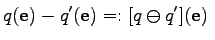(2.33)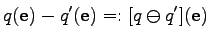(2.34)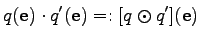(2.35)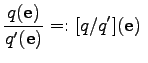(2.36)

It can be seen that the functional component and the argument can be separated so that larger expressions can be formulated using compact formulations. After the structural difference between, for instance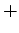and, is observed, it can be easily seen that using the same symbol, namelyfor both notions does not introduce any ambiguity. In order to discriminate between the first order binary operator which is applied on two real or rational numbers and the second order operation which forms a function out of two given functions, the context of the operator symbol has to be considered. If written between values, writing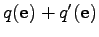the symbol has the meaning of the well known addition operation. When applied like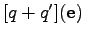the sign has the meaning ofas introduced in 2.33.

The same holds true for the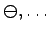notation which compromises the readability of the notation, while no additional information is added.

Michael 2008-01-16